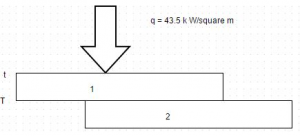# E-PolyLearning

 6. The thermal conductivity and the electrical conductivity of a metal at absolute temperature are related as a. k/σ T b. k/σ c. k σ/T d. k/T

 7. The relation Ϫ² t =0 is referred to as a. Poisson’s equation b. Fourier heat conduction equation c. Solution for transient conduction d. Laplace equation
 9. To effect a bond between two metal plates, 2.5 cm and 15 cm thick, heat is uniformly applied through the thinner plate by a radiant heat source. The bonding must be held at 320 K for a short time. When the heat source is adjusted to have a steady value of 43.5 k W/m², a thermocouple installed on the side of the thinner plate next to source indicates a temperature of 345 K. Calculate the temperature gradient for heat conduction through thinner plate. In the diagram, the upper plate is 2.5 cm thick while the lower is 15 cm thick.a. – 1000 degree Celsius/m b. – 2000 degree Celsius/m c. – 3000 degree Celsius/m d. – 4000 degree Celsius/m